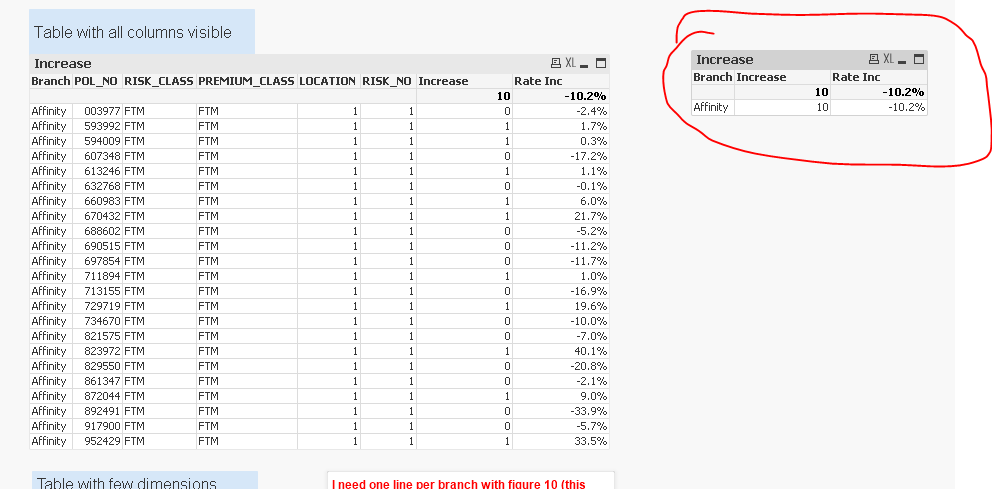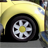# QlikView App Dev

Discussion Board for collaboration related to QlikView App Development.

Announcements
cancel
Showing results for
Did you mean:Contributor II

## Sum of expression

Hi,

I have a table which few dimensions and few expressions on it. Within the table only one dimension column is visible and others are hidden. With applying Sum of Expressions I get total of expression column which is grouping expression with all fields (example given below).

Branch (Dim), Increase (Exp.), RI (Exp.)

6                     , 100%

ABC              , 1                     , 11.2%

ABC              , 0                     , -10.1%

ABC              , 1                     , 5.2%

XYZ              , 0                      , 23.5%

XYZ              , 1                      , 10.2%

CDE              , 1                     , 12.1%

CDE              , 1                     , 10.2%

CDE              , 0                     , 13.1%

GHJ              , 1                     , 11.2%

GHJ              , 0                     , 4.5%

GHJ              , 1                     , 10.6%

The reason why Branch names are repeated is because there are other columns which are hidden. The expression for column Increase is =if (RI <= 2.5/1000,1,0). Please note the example above & below has hypothetical numbers.

I want to show a table with distinct values with expressions as above but still grouping it with other hidden columns, like below.

Branch (Dim), Increase (Exp.), RI (Exp.)

6                     , 100%

ABC              , 2                     , 14.2%

XYZ              , 1                      , 13.5%

CDE              , 2                     , 12.1%

GHJ              , 2                     , 11.2%

The table still needs to split the expression figures by other columns but  it should show one line per Branch.

Is this possible? Any help will be very much appreciated.

1 Solution

Accepted SolutionsMVP

Try this expression for Increase

=Sum(Aggr(if(sum([Rate Increase]) >=2.5/1000,1,0), Branch, POL_NO, RISK_CLASS, PREMIUM_CLASS, LOCATION, RISK_NO))9 RepliesSpecialist III

Hi,

Try with :  aggr(Sum(Increase),Branch)Specialist III

Hi,

Can you attach sample file with hidden columns.

I think we can use Aggregation function to Achieve it.Specialist III

Hi,

You can Use something Like this:

=Aggr(Sum(Expression),Branch,other dimensions( Hidden Dimensions)

Thanks,

MukramMVP

Why add dimension and hide them if they are not serving any purpose?Contributor II
Author

The hidden dimensions are needed to calculate the Increase expressions. The figure 10 shown at the top of Increase column is "Sum of Rows" and not "Expression Total"Contributor II
Author

Hi sample file is attached now. This is showing the problem I am facing and the expected outcome.MVP

Try this expression for Increase

=Sum(Aggr(if(sum([Rate Increase]) >=2.5/1000,1,0), Branch, POL_NO, RISK_CLASS, PREMIUM_CLASS, LOCATION, RISK_NO))Contributor II
Author

Hi Sunny,

thanks a lot for this. this is working in the sample file you returned to me however the same is not working in actual document. Don't know why.Contributor II
Author

Hi Sunny,

The problem was I had put extra Sum due to which it wasn't working, but when I removed the extra Sum it gave me perfect result.

Thanks a ton!!!